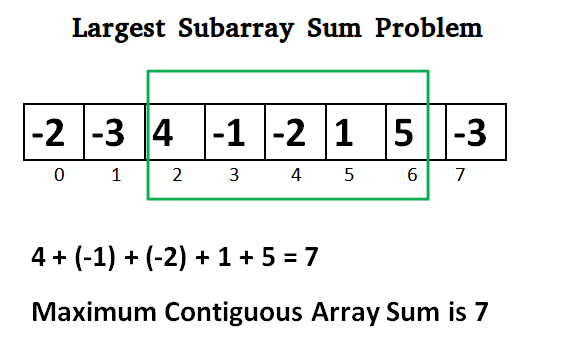# Queation-Given an array arr of N integers. Find the contiguous sub-array with maximum sum.

Input:
The first line of input contains an integer denoting the number of test cases. The description of test cases follows. The first line of each test case contains a single integer denoting the size of array. The second line contains space-separated integers A1, A2, ..., AN denoting the elements of the array.

Output:
Print the maximum sum of the contiguous sub-array in a separate line for each test case.

Constraints:
1 ≤ T ≤ 110
1 ≤ N ≤ 106
-107 ≤ A[i] <= 107

Example:
Input
2
5
1 2 3 -2 5
4
-1 -2 -3 -4
Output
9
-1

Explanation:
Testcase 1:
Max subarray sum is 9 of elements (1, 2, 3, -2, 5) which is a contiguous subarray.image provided by gfg

Solution->

This Question can be solved by using Kadane Algorithm

Here is my solution-